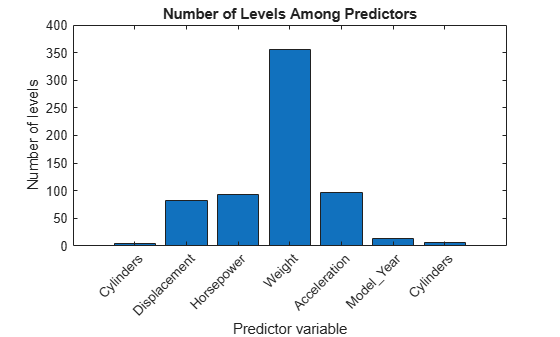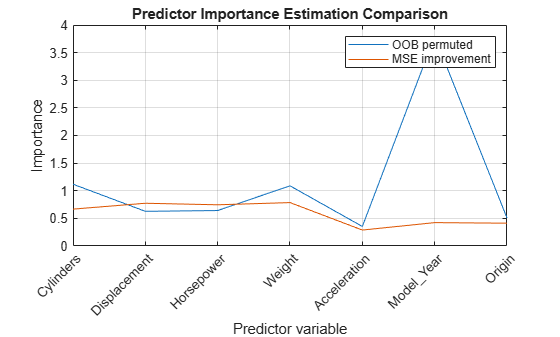Documentation

## Select Predictors for Random Forests

This example shows how to choose the appropriate split predictor selection technique for your data set when growing a random forest of regression trees. This example also shows how to decide which predictors are most important to include in the training data.

Load the `carbig` data set. Consider a model that predicts the fuel economy of a car given its number of cylinders, engine displacement, horsepower, weight, acceleration, model year, and country of origin. Consider `Cylinders`, `Model_Year`, and `Origin` as categorical variables.

```load carbig Cylinders = categorical(Cylinders); Model_Year = categorical(Model_Year); Origin = categorical(cellstr(Origin)); X = table(Cylinders,Displacement,Horsepower,Weight,Acceleration,Model_Year,Origin);```

### Determine Levels in Predictors

The standard CART algorithm tends to split predictors with many unique values (levels), e.g., continuous variables, over those with fewer levels, e.g., categorical variables. If your data is heterogeneous, or your predictor variables vary greatly in their number of levels, then consider using the curvature or interaction tests for split-predictor selection instead of standard CART.

For each predictor, determine the number of levels in the data. One way to do this is define an anonymous function that:

1. Converts all variables to the categorical data type using `categorical`

2. Determines all unique categories while ignoring missing values using `categories`

3. Counts the categories using `numel`

Then, apply the function to each variable using `varfun`.

```countLevels = @(x)numel(categories(categorical(x))); numLevels = varfun(countLevels,X,'OutputFormat','uniform');```

Compare the number of levels among the predictor variables.

```figure bar(numLevels) title('Number of Levels Among Predictors') xlabel('Predictor variable') ylabel('Number of levels') h = gca; h.XTickLabel = X.Properties.VariableNames(1:end-1); h.XTickLabelRotation = 45; h.TickLabelInterpreter = 'none';```The continuous variables have many more levels than the categorical variables. Because the number of levels among the predictors varies so much, using standard CART to select split predictors at each node of the trees in a random forest can yield inaccurate predictor importance estimates. In this case, use the curvature test or interaction test. Specify the algorithm by using the `'PredictorSelection'` name-value pair argument. For more details, see Choose Split Predictor Selection Technique.

### Train Bagged Ensemble of Regression Trees

Train a bagged ensemble of 200 regression trees to estimate predictor importance values. Define a tree learner using these name-value pair arguments:

• `'NumVariablesToSample','all'` — Use all predictor variables at each node to ensure that each tree uses all predictor variables.

• `'PredictorSelection','interaction-curvature'` — Specify usage of the interaction test to select split predictors.

• `'Surrogate','on'` — Specify usage of surrogate splits to increase accuracy because the data set includes missing values.

```t = templateTree('NumVariablesToSample','all',... 'PredictorSelection','interaction-curvature','Surrogate','on'); rng(1); % For reproducibility Mdl = fitrensemble(X,MPG,'Method','Bag','NumLearningCycles',200, ... 'Learners',t);```

`Mdl` is a `RegressionBaggedEnsemble` model.

Estimate the model ${R}^{2}$ using out-of-bag predictions.

```yHat = oobPredict(Mdl); R2 = corr(Mdl.Y,yHat)^2```
```R2 = 0.8744 ```

`Mdl` explains 87% of the variability around the mean.

### Predictor Importance Estimation

Estimate predictor importance values by permuting out-of-bag observations among the trees.

`impOOB = oobPermutedPredictorImportance(Mdl);`

`impOOB` is a 1-by-7 vector of predictor importance estimates corresponding to the predictors in `Mdl.PredictorNames`. The estimates are not biased toward predictors containing many levels.

Compare the predictor importance estimates.

```figure bar(impOOB) title('Unbiased Predictor Importance Estimates') xlabel('Predictor variable') ylabel('Importance') h = gca; h.XTickLabel = Mdl.PredictorNames; h.XTickLabelRotation = 45; h.TickLabelInterpreter = 'none';```Greater importance estimates indicate more important predictors. The bar graph suggests that `Model_Year` is the most important predictor, followed by `Cylinders` and `Weight`. The `Model_Year` and `Cylinders` variables have only 13 and 5 distinct levels, respectively, whereas the `Weight` variable has over 300 levels.

Compare predictor importance estimates by permuting out-of-bag observations and those estimates obtained by summing gains in the mean squared error due to splits on each predictor. Also, obtain predictor association measures estimated by surrogate splits.

```[impGain,predAssociation] = predictorImportance(Mdl); figure plot(1:numel(Mdl.PredictorNames),[impOOB' impGain']) title('Predictor Importance Estimation Comparison') xlabel('Predictor variable') ylabel('Importance') h = gca; h.XTickLabel = Mdl.PredictorNames; h.XTickLabelRotation = 45; h.TickLabelInterpreter = 'none'; legend('OOB permuted','MSE improvement') grid on```According to the values of `impGain`, the variables `Displacement`, `Horsepower`, and `Weight` appear to be equally important.

`predAssociation` is a 7-by-7 matrix of predictor association measures. Rows and columns correspond to the predictors in `Mdl.PredictorNames`. You can infer the strength of the relationship between pairs of predictors using the elements of `predAssociation`. Larger values indicate more highly correlated pairs of predictors.

```figure imagesc(predAssociation) title('Predictor Association Estimates') colorbar h = gca; h.XTickLabel = Mdl.PredictorNames; h.XTickLabelRotation = 45; h.TickLabelInterpreter = 'none'; h.YTickLabel = Mdl.PredictorNames;````predAssociation(1,2)`
```ans = 0.6871 ```

The largest association is between `Cylinders` and `Displacement`, but the value is not high enough to indicate a strong relationship between the two predictors.

### Grow Random Forest Using Reduced Predictor Set

Because prediction time increases with the number of predictors in random forests, a good practice is to create a model using as few predictors as possible.

Grow a random forest of 200 regression trees using the best two predictors only. The default `'NumVariablesToSample'` value of `templateTree` is one third of the number of predictors for regression, so `fitrensemble` uses the random forest algorithm.

```t = templateTree('PredictorSelection','interaction-curvature','Surrogate','on', ... 'Reproducible',true); % For reproducibility of random predictor selections MdlReduced = fitrensemble(X(:,{'Model_Year' 'Weight'}),MPG,'Method','Bag', ... 'NumLearningCycles',200,'Learners',t);```

Compute the ${R}^{2}$ of the reduced model.

```yHatReduced = oobPredict(MdlReduced); r2Reduced = corr(Mdl.Y,yHatReduced)^2```
```r2Reduced = 0.8627 ```

The ${R}^{2}$ for the reduced model is close to the ${R}^{2}$ of the full model. This result suggests that the reduced model is sufficient for prediction.